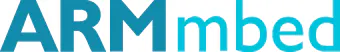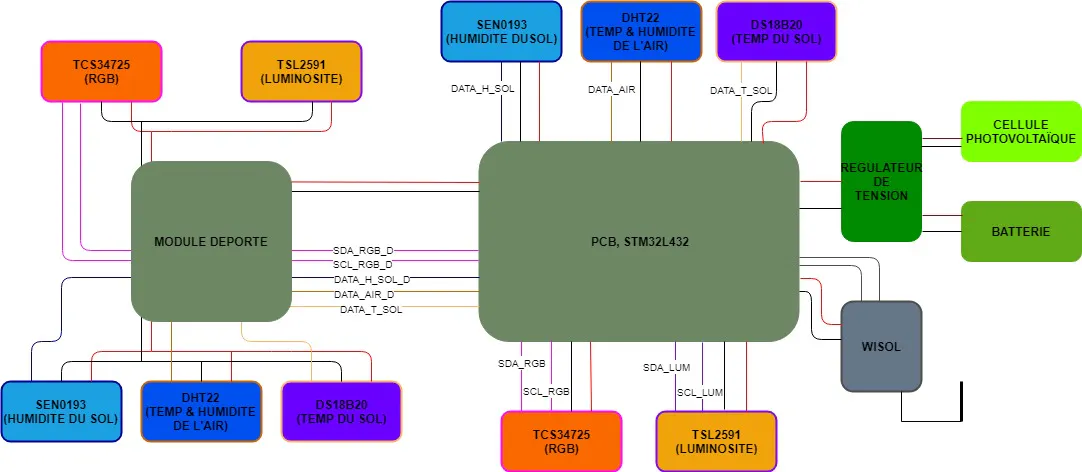Published

# PVSIGHT: Ecosystem Following Device

A device that follows the evolution of an ecosystem through different variables like temperature, humidity and sun exposure.

IntermediateFull instructions providedOver 1 day350## Things used in this project

### Hardware components

 Grove DHT22 Air temperature + Air humidity sensor
×2
 Grove 101990019 (DS18B20) Soil temperature sensor
×2
 Gravity SEN0193 Soil humidity sensor
×2
×2
 ADA 1334 (TCS34725 ) RGB Sensor
×2
 NUCLEO-L432KC STM32 microcontroller
×1
 BRKWS01 Kit Breakout Sigfox + antenna
×1
 Accu Li-Ion 3,7 V 1050 mAh Battery
×1
 DFR0164 USB converter
×1
 SOL2W Solar panel
×1
 Battery charger for solar panel applications
×1
 Tension regulator/ MCP1252/3
×1

### Software apps and online servicesSigfox IoT backend
 SolidWorks 3D Printing Desgin
 PowerBI Data Visualisation
 Eagle PCB designMicrosoft Azure Cloud

### Hand tools and fabrication machinesSoldering iron (generic)3D Printer (generic)Arm mbed

## Custom parts and enclosures

### Main Case

It contains the PCB, the alimentation(excepte the solar panel).
The PCB connects all the sensors that are in the shade and is connected to the secondary case.
It's thiçs case that contains the Nucleo and the Sigfox antenna

### Secondary case

It contains the solar panel, a pcb to treat the data from it's sensors

## Schematics

### Schematic## Code

### main.c

C/C++
```/*
main.c
Author : Ayoub SABRI, Ryan DANEKAS, Antoine ACOLANT, Antoine SOMSAY
Polytech Sorbonne - EISE 4 - 2018/19
*/

/*****************************  LIBRARIES  ***********************************/

#include "mbed.h"
#include "main.h"
#include "DHT.h"
#include "DS1820.h"
#include "TSL2591.h"
#include "TCS3472_I2C.h"
#include "WakeUp.h"

/****************************  CONNECTIONS  **********************************/

DHT             dht_int(DHT_INT_PIN, DHT::DHT22);           // D6
DHT             dht_ext(DHT_EXT_PIN, DHT::DHT22);           // D9

I2C             i2c_int(I2C_INT_SDA,I2C_INT_SCL);           // SDA_1, SCL_1
I2C             i2c_ext(I2C_EXT_SDA,I2C_EXT_SCL);           // SDA_3, SCL_3

TCS3472_I2C     rgb_int(I2C_INT_SDA,I2C_INT_SCL);           // bus I2C 1
TCS3472_I2C     rgb_ext(I2C_EXT_SDA,I2C_EXT_SCL);           // bus I2C 3

DS1820          soil_temp_int(SOIL_TEMP_INT_PIN);           // A2
DS1820          soil_temp_ext(SOIL_TEMP_EXT_PIN);           // A4

AnalogIn        soil_hum_int(SOIL_MOISTURE_INT_PIN);        // SEN0193 - A1
AnalogIn        soil_hum_ext(SOIL_MOISTURE_EXT_PIN);        // SEN0193 - A3

Serial          sigfox(SIGFOX_TX, SIGFOX_RX);
Serial          pc(USBTX, USBRX);                           // UART

/****************************  VARIABLES  ************************************/

int err_int = 0;                        // verify DHT_int sample
int err_ext = 0;                        // verify DHT_ext sample
int x = 0;                              // used in air_temperature processing
int y = 0;                              // used in soil_temperature processing

int lum = 0;                            // used in ReducedLuminosity

uint32_t dataSigfox1_int = 0;           // 32 bit
uint32_t dataSigfox2_int = 0;

uint32_t dataSigfox1_ext = 0;           // 32 bit
uint32_t dataSigfox2_ext = 0;

uint8_t air_temp_int_reduced = 0;       // 8 bit (0.5°C step)
uint8_t air_temp_ext_reduced = 0;       // 8 bit (0.5°C step)

uint8_t soil_temp_int_reduced = 0;      // 8 bit (0.5 °C step)
uint8_t soil_temp_ext_reduced = 0;      // 8 bit (0.5 °C step)

uint8_t air_hum_int_reduced = 0;        // 8 bit (0 to 50% - 1% step)
uint8_t air_hum_ext_reduced = 0;        // 8 bit (0 to 50% - 1% step)

uint8_t soil_hum_int_reduced = 0;       // 8 bit (0 to 50% - 1% step)
uint8_t soil_hum_ext_reduced = 0;

uint8_t lum_int_reduced = 0;            // 8 bit (0 to 255)
uint8_t lum_ext_reduced = 0;

uint8_t rgb_int_red = 0;                // 8 bit (0 to 255)
uint8_t rgb_int_blue = 0;

uint8_t rgb_ext_red = 0;
uint8_t rgb_ext_blue = 0;

/****************************  FUNCTIONS  ************************************/

void  mycallback(void){}

uint8_t GetAirHumidityReduced(int type) {
int air_humidity = 0;
if(type == INT_MEASURE){
air_humidity = (int) dht_int.getHumidity();
}
else if( type == EXT_MEASURE){
air_humidity = (int) dht_ext.getHumidity();
}

if(air_humidity % 2 != 0) { air_humidity ++; }

return air_humidity / 2;
}

uint8_t GetAirTempReduced(int type) {
int air_temperature = 0;
if(type == INT_MEASURE){
air_temperature = (int) dht_int.getTemperature(DHT::CELCIUS) * 10;
}
else if( type == EXT_MEASURE){
air_temperature = (int) dht_ext.getTemperature(DHT::CELCIUS) * 10;
}

x = air_temperature % 10;
if((x > 2) && (x < 8)){
air_temperature = (air_temperature / 10)*10+5;
}
else{
if (x < 5) {air_temperature = (air_temperature/10)*10;}
else {air_temperature =(air_temperature/10)*10+10;}
}
return (int)(air_temperature+300)/5;
}
uint8_t GetSoilTempReduced(int type) {
int soil_temperature = 0;
if(type == INT_MEASURE){
soil_temp_int.convertTemperature(true, DS1820::all_devices);
if(soil_temp_int.unassignedProbe(SOIL_TEMP_INT_PIN))pc.printf("error");
soil_temperature = (int) soil_temp_int.temperature() * 10;
}
else if(type == EXT_MEASURE){
soil_temp_ext.convertTemperature(true, DS1820::all_devices);
if(soil_temp_ext.unassignedProbe(SOIL_TEMP_EXT_PIN))pc.printf("error");
soil_temperature = (int) soil_temp_ext.temperature() * 10;
}

y = soil_temperature % 10;
if((y > 2) && (y < 8)){
soil_temperature = (soil_temperature/10)*10+5;
}
else{
if (y < 5) {soil_temperature = (soil_temperature/10)*10;}
else {soil_temperature =(soil_temperature/10)*10+10;}
}
return (soil_temperature+50)/5;
}

uint8_t GetSoilHumidityReduced(int type){
float Vm = 0.0;
if(type == INT_MEASURE){
}
else if(type == EXT_MEASURE){
}

float soil_humidity = (( 1.0 - Vm ) - 0.23) * 100.0 / 0.4; // 0 to 100% range
return (uint8_t) soil_humidity / 2;
}

uint8_t GetLuminosityReduced(int type){

if(type == INT_MEASURE){
lum_int.getALS();
lum_int.calcLux();
lum = lum_int.lux;
}
else if(type == EXT_MEASURE){
lum_ext.getALS();
lum_ext.calcLux();
lum = lum_ext.lux;
}

return (int) (lum / 16);
}

void printSensorValue_int(){
pc.printf("AT\$SF=%08x%08x\r\n", dataSigfox1_int, dataSigfox2_int);
pc.printf("air temp int:   %d    %.2f\r\n", air_temp_int_reduced, dht_int.getTemperature(DHT::CELCIUS));
pc.printf("soil temp int:  %d    %.2f\r\n", soil_temp_int_reduced, soil_temp_int.temperature());
pc.printf("air hum int:    %d    %.2f\r\n", air_hum_int_reduced, dht_int.getHumidity());
pc.printf("soil hum int:   %d    %d\r\n", soil_hum_int_reduced, soil_hum_int_reduced * 2);
pc.printf("lum int:        %d    %.2f\r\n", lum_int_reduced, lum_int.lux);
pc.printf("rgb red int:    %d\r\nrgb blue int:  %d\r\n\n", rgb_int_red, rgb_int_blue);
}

void printSensorValue_ext() {
pc.printf("AT\$SF=%08x%08x\r\n", dataSigfox1_ext, dataSigfox2_ext);
pc.printf("air temp ext:   %d    %.2f\r\n", air_temp_ext_reduced, dht_ext.getTemperature(DHT::CELCIUS));
pc.printf("soil temp ext:  %d    %.2f\r\n", soil_temp_ext_reduced, soil_temp_ext.temperature());
pc.printf("air hum ext:    %d    %.2f\r\n", air_hum_ext_reduced, dht_ext.getHumidity());
pc.printf("soil hum ext:   %d    %d\r\n", soil_hum_ext_reduced, soil_hum_ext_reduced * 2);
pc.printf("lum ext:        %d    %.2f\r\n", lum_ext_reduced, lum_ext.lux);
pc.printf("rgb red ext:    %d\r\nrgb blue ext:  %d\r\n\n", rgb_ext_red, rgb_ext_blue);
}

void init(){

rgb_int.enablePowerAndRGBC();
rgb_int.setIntegrationTime(100);

rgb_ext.enablePowerAndRGBC();
rgb_ext.setIntegrationTime(100);

lum_int.init();
lum_ext.init();

WakeUp::set_ms(DELAY_SLEEP * 1000);
WakeUp::calibrate();
WakeUp::attach(&mycallback);
}

/*******************************  MAIN  **************************************/

int main() {

// Initalization
pc.format(8,SerialBase::None,1);
pc.baud(9600);

//sigfox.format(8,SerialBase::None,1);
sigfox.baud(9600);

while(1) {

init();
wait_ms(10);

//Air Humidity and Temperature

if (err_int == DHT::SUCCESS) {
air_hum_int_reduced = GetAirHumidityReduced(INT_MEASURE);
air_temp_int_reduced = GetAirTempReduced(INT_MEASURE);
}

if (err_ext == DHT::SUCCESS) {
air_hum_ext_reduced = GetAirHumidityReduced(EXT_MEASURE);
air_temp_ext_reduced = GetAirTempReduced(EXT_MEASURE);
}

// rgb
rgb_int_red = rgb_int.getRedData();
rgb_int_blue = rgb_int.getBlueData();
rgb_ext_red = rgb_ext.getRedData();
rgb_ext_blue = rgb_ext.getBlueData();

// soil temp
soil_temp_int_reduced = GetSoilTempReduced(INT_MEASURE);
soil_temp_ext_reduced = GetSoilTempReduced(EXT_MEASURE);

// soil humidity
soil_hum_int_reduced = GetSoilHumidityReduced(INT_MEASURE);
soil_hum_ext_reduced = GetSoilHumidityReduced(EXT_MEASURE);

//luminosity
lum_int_reduced = GetLuminosityReduced(INT_MEASURE);
lum_ext_reduced = GetLuminosityReduced(EXT_MEASURE);

dataSigfox1_int = ((uint32_t)(air_temp_int_reduced) << 24)    |
((uint32_t)(soil_temp_int_reduced) << 16)   |
((uint32_t)(air_hum_int_reduced) << 8)      |
((uint32_t)(soil_hum_int_reduced));

dataSigfox2_int = ((uint32_t)(lum_int_reduced) << 24) |
((uint32_t)(rgb_int_red) << 16)     |
((uint32_t)(rgb_int_blue) << 8)     |
((uint32_t)(INT_MEASURE));

dataSigfox1_ext = ((uint32_t)(air_temp_ext_reduced) << 24)    |
((uint32_t)(soil_temp_ext_reduced) << 16)   |
((uint32_t)(air_hum_ext_reduced) << 8)      |
((uint32_t)(soil_hum_ext_reduced));

dataSigfox2_ext = ((uint32_t)(lum_ext_reduced) << 24) |
((uint32_t)(rgb_ext_red) << 16)     |
((uint32_t)(rgb_ext_blue) << 8)     |
((uint32_t)(EXT_MEASURE));

sigfox.printf("AT\$SF=%08x%08x\r\n", dataSigfox1_int, dataSigfox2_int);
pc.printf("AT\$SF=%08x%08x\r\n", dataSigfox1_int, dataSigfox2_int);
wait(10);
sigfox.printf("AT\$SF=%08x%08x\r\n", dataSigfox1_ext, dataSigfox2_ext);
pc.printf("AT\$SF=%08x%08x\r\n", dataSigfox1_ext, dataSigfox2_ext);

// Display values
//printSensorValue_int();
//printSensorValue_ext();
//wait_ms(100);

// En veille pendant 20 min

for(int i = 0; i < 20; ++i){
WakeUp::set_ms(DELAY_SLEEP * 1000); // 20 min
wait_ms(10);
deepsleep();
}
}
}
```

### main.h

C/C++
```/* main.h */

#define DHT_INT_PIN D6              //Humidity and temperature
#define DHT_EXT_PIN D9

#define I2C_INT_SDA D0              //I2C1
#define I2C_INT_SCL D1

#define I2C_EXT_SDA D12             //I2C3
#define I2C_EXT_SCL A6

#define SOIL_TEMP_INT_PIN A2        //Soil Temperature
#define SOIL_TEMP_EXT_PIN A4

#define SOIL_MOISTURE_INT_PIN A1    //Ground Humidity
#define SOIL_MOISTURE_EXT_PIN A3

#define SIGFOX_TX D5
#define SIGFOX_RX D4

#define INT_MEASURE 0
#define EXT_MEASURE 1

#define DELAY_SLEEP 60              //  1 min x 20 min
```

### SQL PowerBI

SQL
```/* Module Non Exposé */
SELECT
(((CAST(air_temp as float)*5)-300)/10) as Temp_Air_Int,
(((CAST(soil_temp as float)*5)-50)/10) as Temp_Sol_Int,
(CAST(air_hum as bigint))*2 as Hum_Air_Int,
(CAST(soil_hum as bigint))*2 as Hum_Sol_Int,
CAST(red as bigint) as Rouge_Int,
CAST(blue as bigint) as Bleu_Int,
(CAST(lum as bigint))*15 as Lum_Int,
System.Timestamp as temps
INTO OutputToPowerBI
FROM InputFromIoTHub
WHERE location = '0'

/* Module Exposé */
SELECT
(((CAST(air_temp as float)*5)-300)/10) as Temp_Air_Ext,
(((CAST(soil_temp as float)*5)-50)/10) as Temp_Sol_Ext,
(CAST(air_hum as bigint))*2 as Hum_Air_Ext,
(CAST(soil_hum as bigint))*2 as Hum_Sol_Ext,
CAST(red as bigint) as Rouge_Ext,
CAST(blue as bigint) as Bleu_Ext,
(CAST(lum as bigint))*15 as Lum_Ext,
System.Timestamp as temps
INTO OutputToPowerBI2
FROM InputFromIoTHub
WHERE location = '1'
```

## Credits

### Ryan Danekas

2 projects • 2 followers

### Ayoub Sabri

5 projects • 5 followers
Embedded Systems student at Polytech Sorbonne (Paris)
Thanks to Ayoub Sabri, Antoine Accolant , and Antoine Somsay.